# WIP:LensFlare (ymd:070628)

## Recommended Posts

As this is a work in progress, I post here (instead the plugin section...)

This is where I am on this project: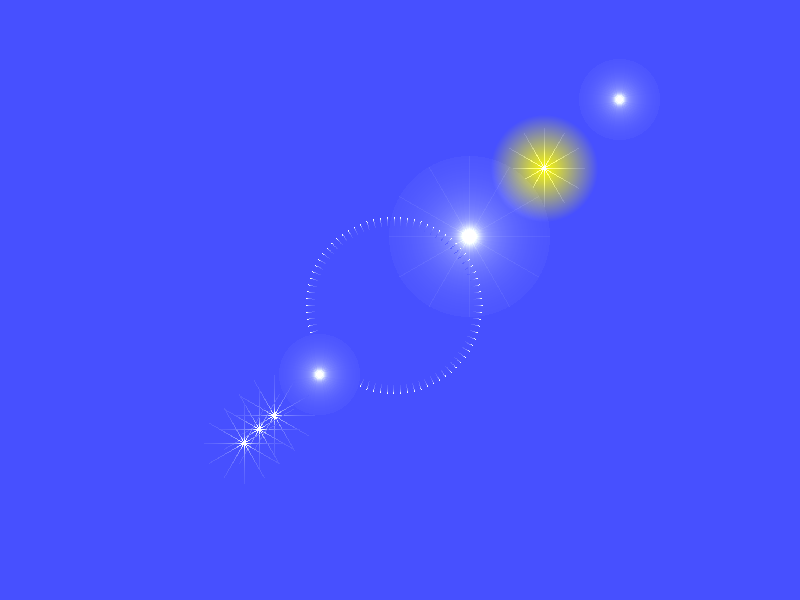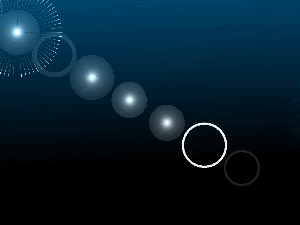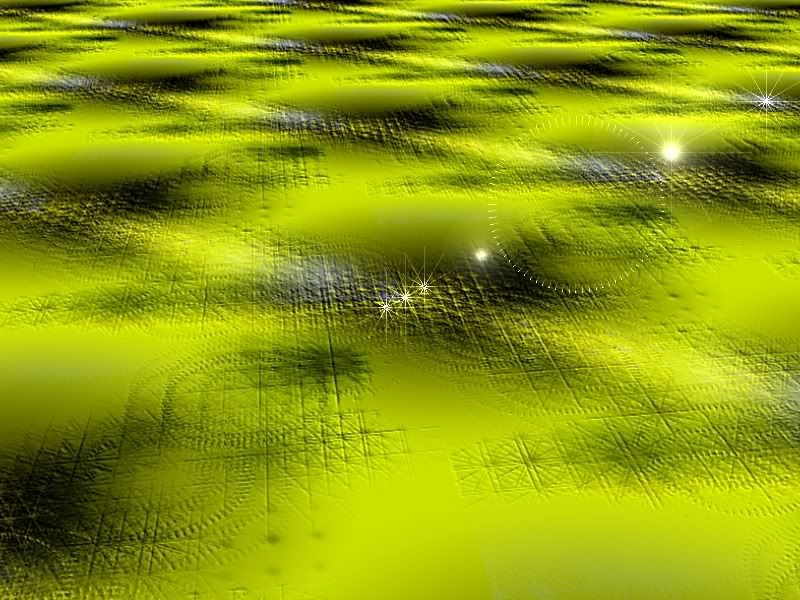__________________________

From a picture, I search for the lightest point of the image.

If there are more than one point, I will retain the first I have found,

meaning the one most on top/left part.

To be sure to choose the source of the light, I add a new layer filled

with black and I spot a single white dot at the right place.

The FXs will be aligned on the line passing through the source of the light

and the center of the image.

__________________________

We could now place at once 8 FXs on this line.

For each FXs we could choose between Flare (circle) or Flake (sparkle).

This are the setting we could define for each FXs:

Type of fx : 0=none, 1=flare, 2=flake (sparkle), 3=both

distance (-/+) from the light

invert the intensity (in -> out / out -> in)

quantity of rays for the flake

//--------------------------------------------------------

// list of settings for each FX (* is number from 1 to 8 )

//--------------------------------------------------------

// F* = Type of fx : 0=none, 1=flare, 2=flake (sparkle), 3=both

// D* = distance (-/+) from the light

// I* = internal radius of the FX

// E* = external radius of the FX

// A* = invert the intensity (in to out / out to in)

// R* = quantity of rays for the flake

//--------------------------------------------------------

int F1 = 1; int D1 =-5; int I1 = 0; int E1 = 10; bool A1 = false; int R1=12;

int F2 = 1; int D2 =15; int I2 = 0; int E2 = 10; bool A2 = false; int R2=12;

int F3 = 3; int D3 = 5; int I3 = 0; int E3 = 20; bool A3 = false; int R3=12;

int F4 = 2; int D4 = 0; int I4 = 0; int E4 = 10; bool A4 = false; int R4=12;

int F5 = 2; int D5 =20; int I5 = 0; int E5 = 10; bool A5 = false; int R5=12;

int F6 = 2; int D6 =18; int I6 = 0; int E6 = 10; bool A6 = false; int R6=12;

int F7 = 2; int D7 =19; int I7 = 0; int E7 = 10; bool A7 = false; int R7=12;

int F8 = 2; int D8 =10; int I8 =20; int E8 = 22; bool A8 = true; int R8=80;

Tip: As each flare/flake is drawn one by one without blending, the last to be drawn is

overlaying the others. To reach a good effect then, sort the FXs like this:

1. Flares

2. Flakes

/Tip

__________________________

This is now some examples of FXs:

Flare / Flake / Both - normal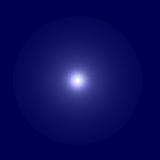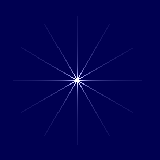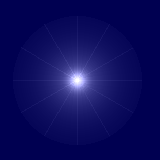Flare / Flake / Both - Inverted intensity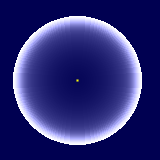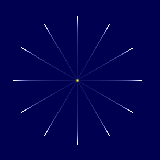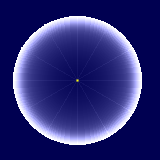Flare / Flake / Both - internal radius >0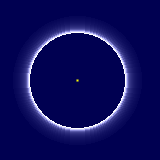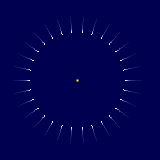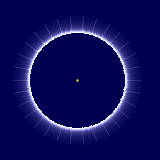On the top of that I've added general factors to affect the distances (Amount1) and the radius (Amount2).

int Amount1 = 150; //[-200,200]Distance factor

// Distance in 1/10th pixel calculated in straight line from the light position.

int Amount2 = 40; //[1,200]Radius factor

// Radius multiplicator in 1/10th pixel.

int Amount3 = 10; //[-255,255]Intensity factor

// Allows to change the intensity for the flakes to make them 'seeable'

// when you choose type of Fx = both.

__________________________

The code for the codelab is well working : good preview, fast enough... but it allows only 3 parameters :!:

It's surprising (in the good way) as it's a so long code (for the codelab IMO)...```int Amount1 = 150; //[-200,200]Distance factor
// Distance in 1/10th pixel calculated in straight line from the light position.

int Amount2 = 40;  //[1,200]Radius factor
// Radius multiplicator in 1/10th pixel.

int Amount3 = 10;  //[-255,255]Intensity factor
// Allows to change the intensity for the flakes to make them 'seeable'
// when you choose type of Fx = both.

//--------------------------------------------------------
// list of settings for each FX  (* is number from 1 to 8)
//--------------------------------------------------------
// F* = Type of fx : 0=none, 1=flare, 2=flake (sparkle), 3=both
// D*  = distance (-/+) from the light
// I*  = internal radius of the FX
// E*  = external radius of the FX
// A*  = invert the intensity (in to out / out to in)
// R*  = quantity of rays for the flake
//--------------------------------------------------------
int F1 = 1; int D1 =-5; int I1 = 0; int E1 = 10; bool A1 = false; int R1=12;
int F2 = 1; int D2 =15; int I2 = 0; int E2 = 10; bool A2 = false; int R2=12;
int F3 = 3; int D3 = 5; int I3 = 0; int E3 = 20; bool A3 = false; int R3=12;
int F4 = 2; int D4 = 0; int I4 = 0; int E4 = 10; bool A4 = false; int R4=12;
int F5 = 2; int D5 =20; int I5 = 0; int E5 = 10; bool A5 = false; int R5=12;
int F6 = 2; int D6 =18; int I6 = 0; int E6 = 10; bool A6 = false; int R6=12;
int F7 = 2; int D7 =19; int I7 = 0; int E7 = 10; bool A7 = false; int R7=12;
int F8 = 2; int D8 =10; int I8 =20; int E8 = 22; bool A8 = true;  int R8=80;
//--------------------------------------------------------

int ProcessStep = 0;
int Value1 = -1;
int Value2 = -1;
int Value3 = -1;
int lightx=100, lighty=100, lightw=0;
float vx=0, vy=0, vd=0, cx=0, cy=0;
void Render(Surface dst, Surface src, Rectangle rect)
{
PdnRegion selectionRegion = EnvironmentParameters.GetSelection(src.Bounds);
Rectangle selection = this.EnvironmentParameters.GetSelection(src.Bounds).GetBoundsInt();

ColorBgra PrimaryColor = (ColorBgra)EnvironmentParameters.PrimaryColor;
ColorBgra SecondaryColor = (ColorBgra)EnvironmentParameters.SecondaryColor;

// Image center
cx = (int)(((selection.Right - selection.Left) / 2)+selection.Left);
cy = (int)(((selection.Bottom - selection.Top) / 2)+selection.Top);

// Test if setting has changed
if (Amount1 != Value1) {Value1=Amount1; ProcessStep = 0;}
if (Amount2 != Value2) {Value2=Amount2; ProcessStep = 0;}
if (Amount3 != Value3) {Value3=Amount3; ProcessStep = 0;}

if (ProcessStep == 0)
{
// Picture analyse to find the light
// pixel closest to color FFFFF (white)
// and backup original image
for(int y = selection.Top; y < selection.Bottom; y++)
{
for (int x = selection.Left; x < selection.Right; x++)
{
dst[x , y] = src[x , y];
int b1 = 0, g1 = 0, r1 = 0, a1 = 0, w1=0;
Accum(ref b1, ref g1, ref r1, ref a1, src[x,y]);
w1 = r1 + g1 + b1 + a1;
if (w1>lightw)
{
lightx = x;
lighty = y;
lightw = w1;
}
}
}
ProcessStep = 1;
}

if (ProcessStep == 1)
{
// Light direction
vx = (float)(lightx - cx);
vy = (float)(lighty - cy);
if(Math.Abs(vx)>Math.Abs(vy)) vd=vx; else vd=vy;
vx /= vd;
vy /= vd;

// Draw the flaRes/flaKes
//DrawFlaKe(dst, src, selectionRegion, int distance, int radiusInt, int radiusExt, int verticies, bool InvertIntensity)
if ((F1==1) || (F1==3)) DrawFlaRe(dst, src, selectionRegion, D1, I1, E1, A1);
if ((F1==2) || (F1==3)) DrawFlaKe(dst, src, selectionRegion, D1, I1, E1, R1, A1);
if ((F2==1) || (F2==3)) DrawFlaRe(dst, src, selectionRegion, D2, I2, E2, A2);
if ((F2==2) || (F2==3)) DrawFlaKe(dst, src, selectionRegion, D2, I2, E2, R2, A2);
if ((F3==1) || (F3==3)) DrawFlaRe(dst, src, selectionRegion, D3, I3, E3, A3);
if ((F3==2) || (F3==3)) DrawFlaKe(dst, src, selectionRegion, D3, I3, E3, R3, A3);
if ((F4==1) || (F4==3)) DrawFlaRe(dst, src, selectionRegion, D4, I4, E4, A4);
if ((F4==2) || (F4==3)) DrawFlaKe(dst, src, selectionRegion, D4, I4, E4, R4, A4);
if ((F5==1) || (F5==3)) DrawFlaRe(dst, src, selectionRegion, D5, I5, E5, A5);
if ((F5==2) || (F5==3)) DrawFlaKe(dst, src, selectionRegion, D5, I5, E5, R5, A5);
if ((F6==1) || (F6==3)) DrawFlaRe(dst, src, selectionRegion, D6, I6, E6, A6);
if ((F6==2) || (F6==3)) DrawFlaKe(dst, src, selectionRegion, D6, I6, E6, R6, A6);
if ((F7==1) || (F7==3)) DrawFlaRe(dst, src, selectionRegion, D7, I7, E7, A7);
if ((F7==2) || (F7==3)) DrawFlaKe(dst, src, selectionRegion, D7, I7, E7, R7, A7);
if ((F8==1) || (F8==3)) DrawFlaRe(dst, src, selectionRegion, D8, I8, E8, A8);
if ((F8==2) || (F8==3)) DrawFlaKe(dst, src, selectionRegion, D8, I8, E8, R8, A8);
ProcessStep = 2;
}
}

private void DrawFlaRe(Surface dst, Surface src, PdnRegion selectionRegion, int distance, int radiusInt, int radiusExt, bool InvertIntensity)
{
float Intensity = 0;
int x,y;
float fx = (float)(lightx - vx * distance * Value1 / 10f);
float fy = (float)(lighty - vy * distance * Value1 / 10f);
int Rint = (int)(radiusInt * (float)Value2 / 10f);
int Rext = (int)(radiusExt * (float)Value2 / 10f);
if (Rext < Rint)
{
Rint = (int)(radiusInt * (float)Value2 / 10f);
Rext = (int)(radiusExt * (float)Value2 / 10f);
}
if (Rext == Rint) Rint = Rext -1;

int b1 = 0, g1 = 0, r1 = 0, a1 = 0;

// Calculation for one quarter and plotting 4x
for (int rY = 0; rY <= Rext ; rY += 1)
{
for (int rX = 0; rX <= Rext ; rX += 1)
{
int tmpR = (int)(0.5f + Math.Sqrt(rX * rX + rY * rY));
if ((tmpR >= Rint) &&(tmpR <= Rext))
{
float wR = (float)(tmpR - Rint) / (float)(Rext - Rint) * 100f;
float wRi = (float)(100 - wR);
if (InvertIntensity) Intensity = (float)(Math.Exp(-(wRi)*(wRi)*0.006) + Math.Exp(-(wRi)*0.03)) / 2.0f;
else Intensity = (float)(Math.Exp(-wR*wR*0.006) + Math.Exp(-wR*0.03)) / 2.0f;

x = (int)(fx + rX);
y = (int)(fy + rY);
if (selectionRegion.IsVisible(x, y))
{
byte col = (byte)(255 * Intensity);
b1 = col; g1 = col; r1 = col; a1 = col;
Accum(ref b1, ref g1, ref r1, ref a1, src.GetBilinearSample(x,y));
dst[x, y] = ColorBgra.FromBgra(Utility.ClampToByte(b1), Utility.ClampToByte(g1), Utility.ClampToByte(r1),Utility.ClampToByte(a1));
}
x = (int)(fx - rX);
y = (int)(fy + rY);
if (selectionRegion.IsVisible(x, y))
{
byte col = (byte)(255 * Intensity);
b1 = col; g1 = col; r1 = col; a1 = col;
Accum(ref b1, ref g1, ref r1, ref a1, src.GetBilinearSample(x,y));
dst[x, y] = ColorBgra.FromBgra(Utility.ClampToByte(b1), Utility.ClampToByte(g1), Utility.ClampToByte(r1),Utility.ClampToByte(a1));
}
x = (int)(fx - rX);
y = (int)(fy - rY);
if (selectionRegion.IsVisible(x, y))
{
byte col = (byte)(255 * Intensity);
b1 = col; g1 = col; r1 = col; a1 = col;
Accum(ref b1, ref g1, ref r1, ref a1, src.GetBilinearSample(x,y));
dst[x, y] = ColorBgra.FromBgra(Utility.ClampToByte(b1), Utility.ClampToByte(g1), Utility.ClampToByte(r1),Utility.ClampToByte(a1));
}
x = (int)(fx + rX);
y = (int)(fy - rY);
if (selectionRegion.IsVisible(x, y))
{
byte col = (byte)(255 * Intensity);
b1 = col; g1 = col; r1 = col; a1 = col;
Accum(ref b1, ref g1, ref r1, ref a1, src.GetBilinearSample(x,y));
dst[x, y] = ColorBgra.FromBgra(Utility.ClampToByte(b1), Utility.ClampToByte(g1), Utility.ClampToByte(r1),Utility.ClampToByte(a1));
}
}
}
}
}

private void DrawFlaKe(Surface dst, Surface src, PdnRegion selectionRegion , int distance, int radiusInt, int radiusExt, int verticies, bool InvertIntensity)
{
float th = (float)(2.0f * Math.PI / verticies);
float Intensity = 0;
int b1 = 0, g1 = 0, r1 = 0, a1 = 0;

float fx = (float)(lightx - vx * distance * Value1 / 10f);
float fy = (float)(lighty - vy * distance * Value1 / 10f);
int Rint = (int)(radiusInt * (float)Value2 / 10f);
int Rext = (int)(radiusExt * (float)Value2 / 10f);
if (Rext < Rint)
{
Rint = (int)(radiusInt * (float)Value2 / 10f);
Rext = (int)(radiusExt * (float)Value2 / 10f);
}
if (Rext == Rint) Rint = Rext -1;

for (int R =  Rint; R <= Rext ; R += 1)
{
float wR = (float)(R - Rint) / (float)(Rext - Rint) * 100f;
float wRi = (float)(100 - wR);
if (InvertIntensity) Intensity = (float)(Math.Exp(-(wRi)*(wRi)*0.006) + Math.Exp(-(wRi)*0.03)) / 2.0f;
else Intensity = (float)(Math.Exp(-wR*wR*0.006) + Math.Exp(-wR*0.03)) / 2.0f;
for (float P =  (float)(- Math.PI); P <= Math.PI; P += th)
{
int x = (int)((float)(R *  Math.Cos(P) + fx ));
int y = (int)((float)(R *  Math.Sin(P) + fy ));
if (selectionRegion.IsVisible(x, y))
{
byte col = Utility.ClampToByte(255 * Intensity + Value3);
b1 = col; g1 = col; r1 = col; a1 = col;
//Accum(ref b1, ref g1, ref r1, ref a1, dst.GetBilinearSample(x,y));
Accum(ref b1, ref g1, ref r1, ref a1, src.GetBilinearSample(x,y));
dst[x, y] = ColorBgra.FromBgra(Utility.ClampToByte(b1), Utility.ClampToByte(g1), Utility.ClampToByte(r1),Utility.ClampToByte(a1));
}
}
}
}

private void Accum(ref int b, ref int g, ref int r, ref int a, ColorBgra col)
{
b += col.B; g += col.G; r += col.R; a += col.A;
}
```

__________________________

Next steps...

create the full UI for the 8 FXs...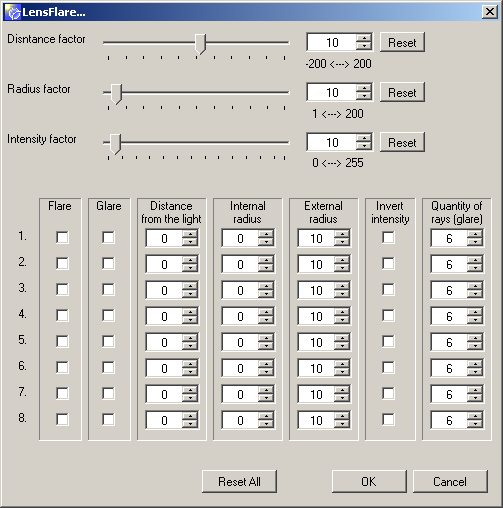But now, I've trouble with the preview :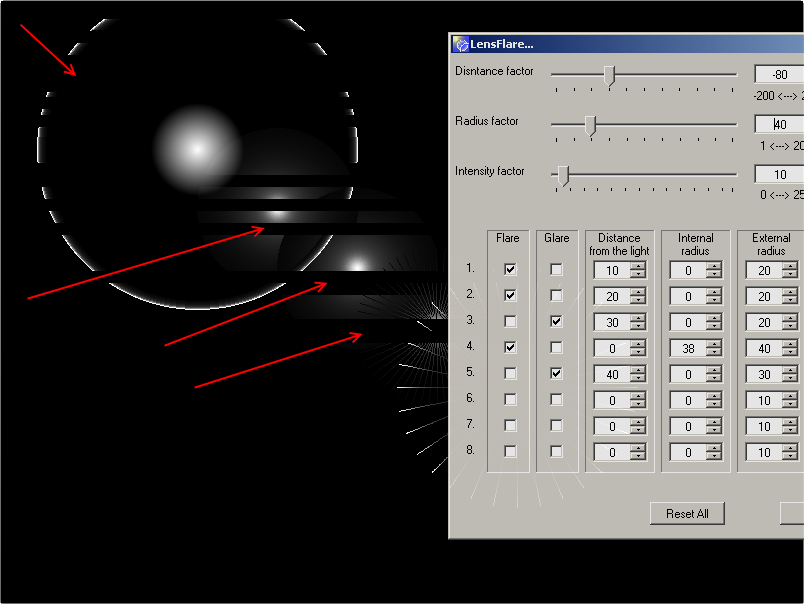and also the rendering,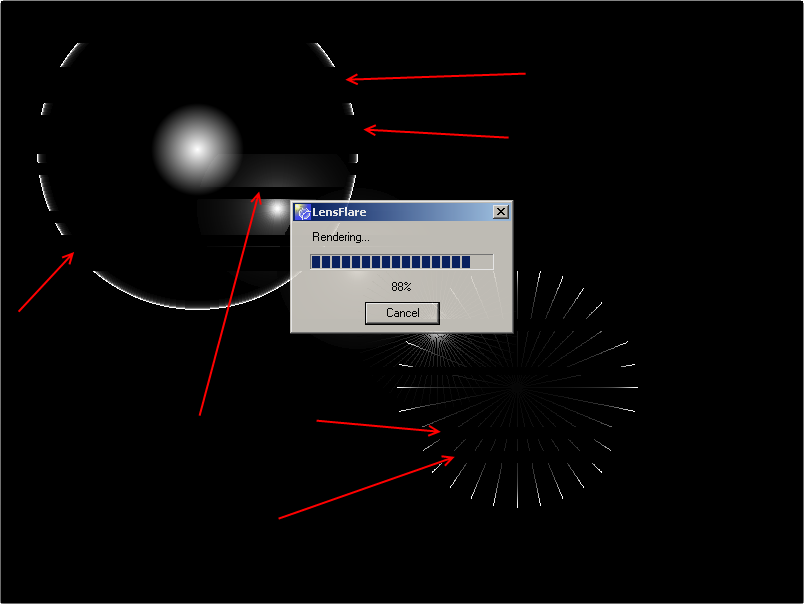Hopefully (could I say that?) the result is correct!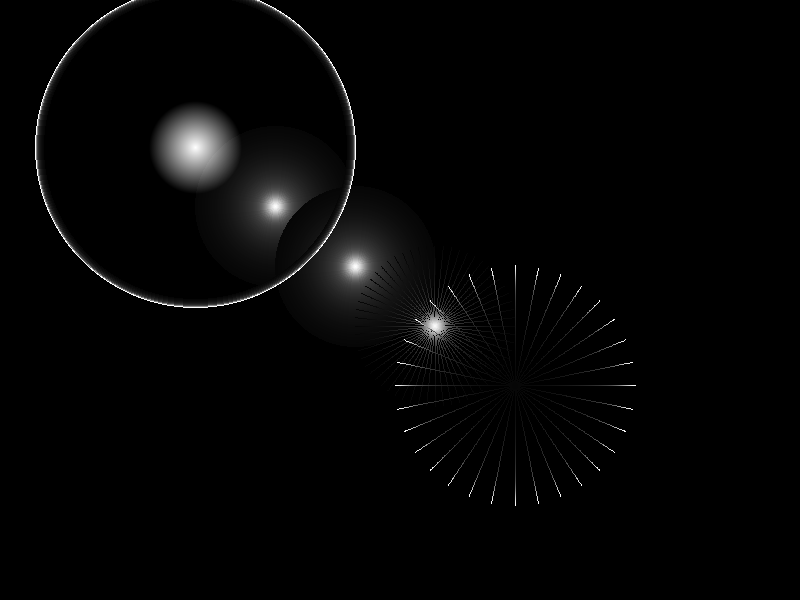I'm scared I will have to use a table (as for the GenTree) to avoid this problem. But this scares me even more

as we should use this lens flare plugin with big images to have a good result... CPU,Memory,...I've other troubles with the UI. For now I can't recall the settings for the FXs. They are each time reset to default!Suggestions are welcome!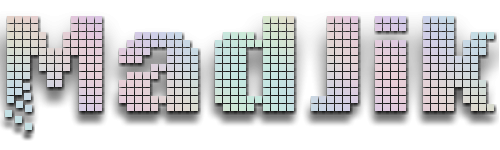My DeviantArt | My Pictorium | My Plugins | Donate via Paypal

##### Share on other sites

I can screenshot the flare effect from illustrator, for reference, if you want...

Yes thanks...My DeviantArt | My Pictorium | My Plugins | Donate via Paypal

##### Share on other sites

Now that's complicated! Cant wait until it's ready##### Share on other sites

Really amazed at your screen shots! Truly beautiful.

Can't wait to try the plugin when it's ready.

Glad you're such a creative programmer.

Regard,

David

##### Share on other sites

I can screenshot the flare effect from illustrator, for reference, if you want...

Yes thanks...

'kay...itlss be tommorrow AM...don't have it at home.

##### Share on other sites

I have a feeling this will be almost as popular as Shape 3D##### Share on other sites

you got the same rendering issues in the preview I have with the align plugin.

anyway I think its complicated of an interface. for a lens flare, it should be simple, in photoshop there are 4 types and a brightness control for each type.

you can download a psd which you can open in pdn with the psd file type plugin. I made all 4 types, one one each layer. I think the 50-300mm zoom is the one most people recognize and the one you are trying to do.

This is lovely.

##### Share on other sites

• 2 weeks later...
I can screenshot the flare effect from illustrator, for reference, if you want...

Yes thanks...

'kay...itlss be tommorrow AM...don't have it at home.

I downloaded GIMP due to personal interest. It came with two lens flare effects an i made a few pics of them. I don't know if they are useful, but I'll share them now.

The normal lens flare:It looks pretty realistic.

The second one is called "GFlare" and provides a lot of options. You can use it to render an alternative lens flare or to do things that look close to MadJik's images above.Now I will show you how it looks using both of them not on a black background. So i got a pic of a weird creature from a short youtube-clip.

Original:Lens flare:And finally GFlare, using other settings::? Hope it helps :?

After spending time with GIMP I gained deep understanding why there are attempts to port PDN to Linux. The only purpose of this UI seems to be to drive people crazy enough to look like the strange being above.

##### Share on other sites

These are the flares from Adobe Illustrator: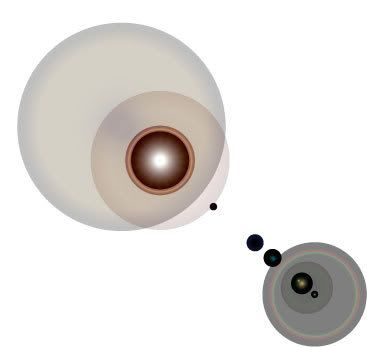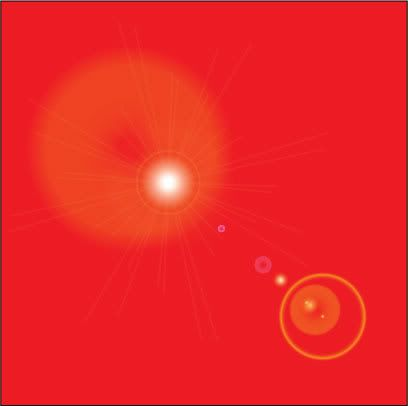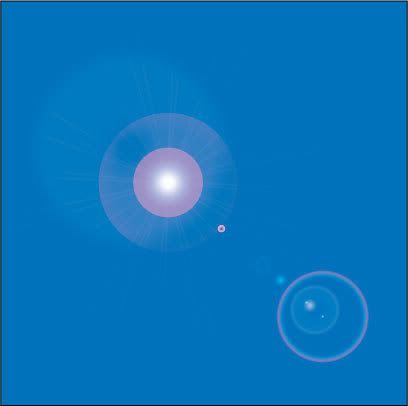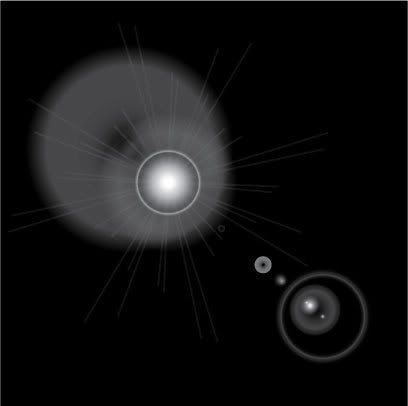They interact differently with different colors. Blending modes?

A transparent .PNG: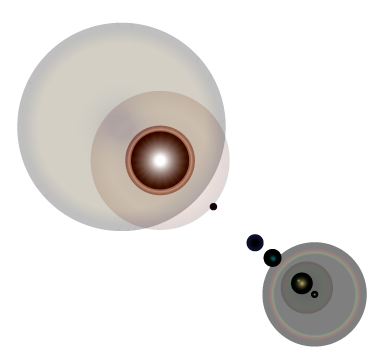The Doctor: There was a goblin, or a trickster, or a warrior... A nameless, terrible thing, soaked in the blood of a billion galaxies. The most feared being in all the cosmos. And nothing could stop it, or hold it, or reason with it. One day it would just drop out of the sky and tear down your world.
Amy: But how did it end up in there?
The Doctor: You know fairy tales. A good wizard tricked it.
River Song: I hate good wizards in fairy tales; they always turn out to be him.

##### Share on other sites

I should also add that the AI interface is in a click-drag-click format (in CS2, at least) - the first click selects the location, the drag sets the angle and size of the main bright spot, and the second click sets the direction of the flare, the location of the secondary bright spot, and the sizes and directions of some of the big, background circles in the main bright spot. I realize that this can't be programmed in the UI, but maybe this will help in programming.

EDIT: Oh, and what you have so far looks great!

The Doctor: There was a goblin, or a trickster, or a warrior... A nameless, terrible thing, soaked in the blood of a billion galaxies. The most feared being in all the cosmos. And nothing could stop it, or hold it, or reason with it. One day it would just drop out of the sky and tear down your world.
Amy: But how did it end up in there?
The Doctor: You know fairy tales. A good wizard tricked it.
River Song: I hate good wizards in fairy tales; they always turn out to be him.

##### Share on other sites

do I copy and paste somewhere?

I'm going to give this a whirl this week.

chow

##### Share on other sites

GIMP flares look better anyway...sorry I dropped the ball on posting the illustrator ones (work and kids intervened)

##### Share on other sites

do I copy and paste somewhere?

I'm going to give this a whirl this week.

chow

1. Select the text of the code above, copy it (Ctrl+C)

2. In codelab, click somewhere in the text area. Select all the text (Ctrl+A). Then paste the code (Ctrl+V)

3. Do the change in the parameters...My DeviantArt | My Pictorium | My Plugins | Donate via Paypal

##### Share on other sites

And thanks all for the samples you've provided...

That should help!My DeviantArt | My Pictorium | My Plugins | Donate via Paypal

##### Share on other sites

Here are screenshots of GIMP's UI:

Lens flare (FlareFX):The cross is moveable.

GFlare's interface is more complicated, as there are more options. I will not provide all of the settings, it's just too much.##### Share on other sites

I think the FlareFX UI is much better just for the simplicity of it. Maybe a few options for like length of overall flare, width of overall flare and possibly colour scheme or simply a colour palette like the one in the Drop Shadow plugin.

I believe (and this is no dig against what you already have or are doing) that too many options immediately hits the user with a sudden sense of "whoa?!" and it may become a daunting experience each time they want to utilize the plugin. But this is just my opinion and I will be happy with whatever comes out of the wood works.

##### Share on other sites

I agree with myrddin on the whole "whoa" factor. It might be useful to have a "simple/advanced" layout, where a common (i.e. your favorite) set of detailed settings is preset (and not alterable) in the simple view (but basic things like direction and size can be changed), and everything is adjustable in the advanced view.

That way, nobody gets scared off, and power users can tinker with it to their heart's delight.

##### Share on other sites

You are not making my (programer's) life easy...

I will try to do my best on this UI...(I'm not yet accurate with C# so...)My DeviantArt | My Pictorium | My Plugins | Donate via Paypal

##### Share on other sites

Yeah, the GFlare UI is just awful. On the other hand, FlareFX provides to less options, and indeed there should be a colour picker or something like that.

##### Share on other sites

MadJik - would applying a UI like the Fast Zoom Blur (as far as direction) be easiest? I think that UI is phenomenal.

BTW, at the time I'm posting this, your post count is 1138. The sci-fi geek in me giggles.

The Doctor: There was a goblin, or a trickster, or a warrior... A nameless, terrible thing, soaked in the blood of a billion galaxies. The most feared being in all the cosmos. And nothing could stop it, or hold it, or reason with it. One day it would just drop out of the sky and tear down your world.
Amy: But how did it end up in there?
The Doctor: You know fairy tales. A good wizard tricked it.
River Song: I hate good wizards in fairy tales; they always turn out to be him.

##### Share on other sites

BTW, at the time I'm posting this, your post count is 1138. The sci-fi geek in me giggles.

Haha.THX.

That's funny.

##### Share on other sites

Are you talk about THX1138? GLucas?My DeviantArt | My Pictorium | My Plugins | Donate via Paypal

##### Share on other sites

Yep. The movie itself, as well as all the references to it in various movies since.

George Lucas seems a bit addicted to it... http://en.wikipedia.org/wiki/THX_1138#R ... ther_media

The Doctor: There was a goblin, or a trickster, or a warrior... A nameless, terrible thing, soaked in the blood of a billion galaxies. The most feared being in all the cosmos. And nothing could stop it, or hold it, or reason with it. One day it would just drop out of the sky and tear down your world.
Amy: But how did it end up in there?
The Doctor: You know fairy tales. A good wizard tricked it.
River Song: I hate good wizards in fairy tales; they always turn out to be him.

## Join the conversation

You can post now and register later. If you have an account, sign in now to post with your account.×   Pasted as rich text.   Paste as plain text instead

Only 75 emoji are allowed.

×   Your previous content has been restored.   Clear editor

×   You cannot paste images directly. Upload or insert images from URL.

×
×